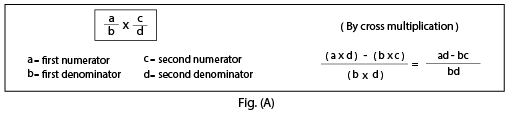Subtraction of fractions with different denominator

## Objective

To subtract a smaller fraction from a greater fraction with different denominators.

## Pre-requisite Knowledge

• Concept of subtraction of natural numbers.
• Concept of fractions.

## What is a fraction?

A fraction is a numerical value that represents the parts of the whole.

## Parts of a fraction

A fraction consists of two parts, namely the numerator and the denominator. The upper part of the fraction is called the numerator, and the lower part of the fraction is called the denominator. For example, 4/7 is a fraction. Here, 4 is the numerator and 7 is the denominator.

## Unlike fractions

• Fractions with different denominators are called unlike fractions.
• Thus, 1/5, 2/7, 3/2, and 8/6 are all unlike fractions.

## Subtraction of unlike fractions• Subtraction of unlike fractions means subtracting fractions with the different denominator values.
• Follow the below steps to subtract the unlike fractions.
• Step 1: Multiplication of the first denominator (b) by the second denominator (d) (refer to Fig. (A)).
• Step 2: Subtract the multiplication of the first denominator (b) by the second numerator (c) from the multiplication of the first numerator (a) by the second denominator (d) (refer to Fig. (A)).
• Step 2: If required, simplify the fraction.
• Example 1: Subtract 2/3 from 5/7.
• Solution:
• Given: (5/7) - (2/3) Here, the denominator values are the different.
• Now, multiply the denominator values.
• (5/7) - (2/3) = ((5 * 3) - (7 * 2))/(7 * 3)
• (5/7) - (2/3) = (15 - 14)/21